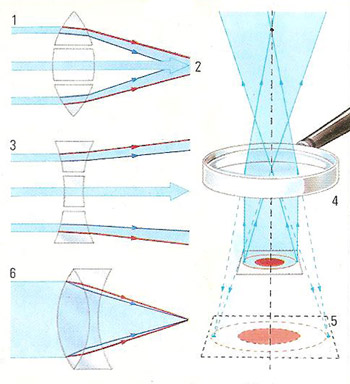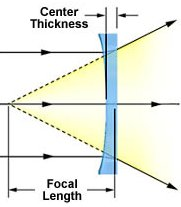A# lensFig 2. A convex lens (1) focuses parallel rays of light on a single point (2), whereas a concave lens causes the rays to diverge. A magnifying glass (4) is a convex lens that alters the path of light rays so that they appear to come from a larger image (5) than is the actual case. Cameras use a combination of convex and concave lenses (6) to focus light without separation of the colors of the spectrum.Fig 3. Ray diagrams showing different ways in which a magnifying glass can be used: (A) as a simple microscope; (B) to form a real image; (C) as a burning glass.

A lens is a piece of transparent material having at least one curved surface and which is used to produce an image or otherwise focus light by refraction (Fig 1). Lenses are found in cameras, glasses, microscopes, telescopes, and other optical instruments. The typical thin lens is formed from a glass disk, though crystalline minerals and molded plastics are also used and, as with spectacle lenses, shapes other than circular are quite common.Fig 1. Light passing through a lens is actually bent at each surface. However, in simple ray diagrams it is possible to disregard this and draw all the bending at a single line (the principal plane) running down the center of the lens.

The principal axis of a lens is the imaginary line perpendicular to its surface at its center. Lenses which are thicker in the middle than at the edges focus a parallel beam of light traveling along the principal axis at the principal focus, a point on the axis on the far side of the lens from the light source. Such lenses are converging lenses. The distance between the principal focus and the center of the lens is known as the focal length of the lens; its focal power is the reciprocal of the focal length and is expressed in diopters (m–1).

A lens thicker at its edges than in the middle spreads out a parallel beam of light passing through along its principal axis as if it were radiating from a virtual focus one focal length from the lens center on the same side as the source. Such a lens is a diverging lens.

Lens surfaces may be either inward curving (concave), outward bulging (convex), or flat (plane) and it is the combination of the properties of the two surfaces which determines the focal power of the lens (Fig 2).

In general, images of objects produced using single thin lenses suffer from various defects including spherical and chromatic aberration, coma (in which peripheral images of points are disturbed into pear-shaped spots), and astigmatism. The effects of these are minimized by designing compound lenses in which simple lenses of different shapes and refractive indices are combined. Achromatic lenses reduce chromatic aberration; aplanatic lenses reduce this and coma, and astigmatic lenses combat astigmatism.

A lensmeter is an instrument used to measure the refractive properties of lenses.

## Plano-concave lensFigure 4. plano-concave lens.

A plano-concave lens is a negative (diverging) spherical lens with one concave surface and one flat (plano) surface.

## Plano-convex lensFigure 5. plano-concave lens.

A plano-concave lens is a positive (converging) spherical lens with one convex surface and one flat (plano) surface.

## Doublet

A doublet is a compound lens made from two elements. The elements may be cemented together (forming a cemented doublet) or separated by an air space (forming a separated doublet). The most common doublet is the achromatic lens, consisting of a crown glass and a flint glass element chosen so that the indices of refraction of two separated wavelengths (one red, one blue) are equal.

## Magnifying glass

A magnifying glass is a converging (convex) lens used to form an enlarged image of an object (Fig 3). A magnifying glass generally has handle attached to a circular clip holding the lens.

In normal use, the object is held within the focal length of the lens and an enlarged, upright virtual image is seen through the lens. A magnifying glass can also be used to form a real but inverted image of an object if the object is placed outside the focal length of the lens. A large converging lens can also be used as a burning glass, focusing light and heat from the Sun.